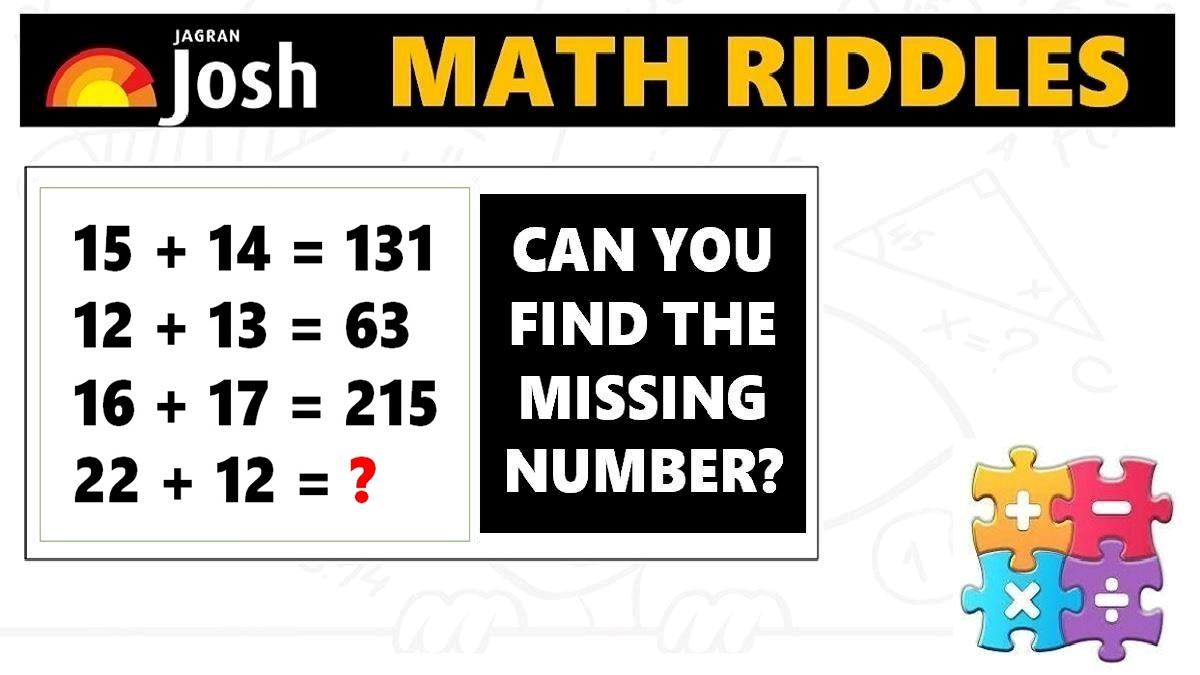# Math Riddles with Answers: Math Puzzles To Increase Your Brain Power

Math Riddles Challenge: Check out these math riddles for geniuses. Find out the missing numbers for each puzzle in less than 1 minute. Check solutions to learn easy way to solve math puzzles.Math Riddles: Are you preparing for competitive exams and looking to ace the quantitative or logical reasoning sections? Well, check out these puzzles that will help you learn maths tricks to solve tricky mathematical problems and expand your knowledge base of maths concepts. Well, the challenge is to solve the math riddles in less than 1 minute each.

Explanation:

## Tell us in comments: Did you solve this mathematics puzzle in less than 1 minute?

Check out more math puzzles!